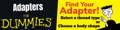If you have the part number then try using our search bar to find it quicker!

(IE. 1404)

03 = 3/16"
04 = 1/4"
05 = 5/16"
06 = 3/8"

12 = 3/4"
14 = 7/8"
16 = 1"

24 = 1-1/2"
32 = 2"